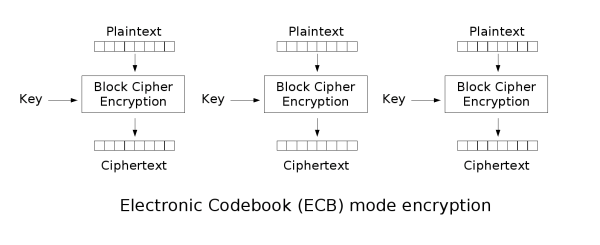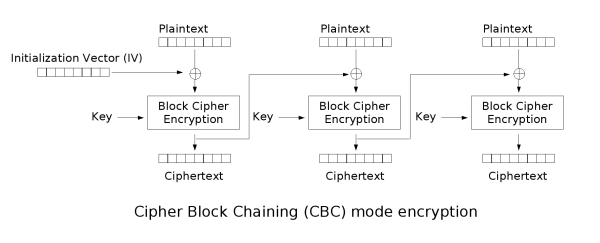# Using encryption and decryption in Java

## Instructions

In project development, in order to ensure the security of data and user privacy, we usually encrypt key information, this article details how to use hutool in java language to quickly encrypt and decrypt data, hope you can help.

If your project is built on Maven, you can introduce Hutool through pom to use the encryption and decryption function.

``````<dependency>
<groupId>cn.hutool</groupId>
<artifactId>hutool-crypto</artifactId>
<version>5.7.15</version>
</dependency>
``````

If your project is not built based on maven, you can also directly download the jar package to use.

`Download Links`: https://repo1.maven.org/maven2/cn/hutool/hutool-all/5.8.0.M1

## Symmetric versus asymmetric encryption

### Symmetric encryption

#### Encryption Algorithm

• An encryption method that uses a single-key cryptosystem where the same key can be used as both encryption and decryption of a message is called symmetric encryption, also known as single-key encryption.
• Common encryption algorithms
• `DES(Data Encryption Standard)` : Data Encryption Standard, a block algorithm using key encryption, was established as a Federal Information Processing Standard (FIPS) by the National Bureau of Standards of the U.S. federal government in 1977 and authorized for use in unclassified government communications, and the algorithm has since spread widely internationally.
• `AES (Advanced Encryption Standard)` : Advanced Encryption Standard. Also known as Rijndael cryptography in cryptography, it is a block encryption standard adopted by the U.S. federal government. This standard is used to replace the original DES, which has been analyzed by many parties and is widely used around the world.
• Features
• Fast encryption speed, can encrypt large files
• Ciphertext reversible, if the key file is leaked, the data will be exposed
• After encryption, the encoding table can not find the corresponding characters, resulting in garbled code, usually used in combination with Base64

#### encryption mode

• `ECB(Electronic codebook)` : Electronic codebook. The message to be encrypted is divided into several blocks according to the block size of the block cipher, and each block is encrypted independently.• Advantages: data can be processed in parallel
• Disadvantage: the same original text generates the same ciphertext, which does not protect the data well
• Simultaneous encryption, the original text is the same and the encrypted ciphertext is also the same
• `CBC(Cipher-block chaining)` : Cipher-block linking. Each plaintext block is first heterogeneous with the previous ciphertext block before encryption, and each ciphertext block depends on all the plaintext blocks before it• Advantage: the same original text generates different ciphertexts
• Disadvantage: serial processing of data

When data needs to be processed by block, and the data length does not meet the block processing requirements, the block length is filled according to certain rules

• `NoPadding` no padding
• In `DES` encryption algorithm, the length of the original text must be an integer multiple of `8byte`.
• Under `AES` encryption, the original text length must be an integer multiple of `16byte`.
• `PKCS5Padding`
• The size of the data block is 8 bits, if it is not enough, it will be filled

Tips: By default, the encryption mode and padding mode are: `ECB/PKCS5Padding`. If you use `CBC` mode, you need to add the parameter initialization vector `IV`

### DES and AES sample code

``````public class SymmetricCryptoTest {
@Test
public void des() {
String text = "HelloWorld";
// key: in DES mode, the key must be 8 bits
String key = "12345678";
// iv: offset, not required in ECB mode, must be 8 bits in CBC mode
String iv = "12345678";
String encrypt = des.encryptBase64(text);
System.out.println(encrypt);
String decrypt = des.decryptStr(encrypt);
System.out.println(decrypt);
}
@Test
public void aes() {
String text = "HelloWorld";
// key：AES mode, the key must be 16 bits
String key = "1234567812345678";
// iv: offset, not required in ECB mode, must be 16 bits in CBC mode
String iv = "1234567812345678";
// Encryption and Base transcoding
String encrypt = aes.encryptBase64(text);
System.out.println(encrypt);
// Decrypt to string
String decrypt = aes.decryptStr(encrypt);
System.out.println(decrypt);
}
}
``````

## Asymmetric Encryption

### Introduction

• Asymmetric encryption algorithms are also known as modern encryption algorithms.
• Asymmetric encryption is the cornerstone of computer communication security, ensuring that encrypted data cannot be broken.
• Unlike symmetric encryption algorithms, asymmetric encryption algorithms require two keys: a public key `(publickey)` and a private key `(privatekey)`
• The public key and the private key are a pair
• If the data is encrypted with the public key, it can only be decrypted with the corresponding private key.
• If the data is encrypted with a private key, it can only be decrypted with the corresponding public key.
• Because encryption and decryption use two different keys, this algorithm is called an asymmetric encryption algorithm.
• Features
• Different keys are used for encryption and decryption
• Slower processing of data, because of high security level
• Common algorithms
• RSA
• ECC

### `RSA` example

``````public class AsymmetricCryptoTest {
/**
* private key and public key
*/
private static String privateKey;
private static String publicKey;
private static String encryptByPublic;
/**
* Generate public and private keys
*/
@BeforeAll
public static void genKey() {
KeyPair pair = SecureUtil.generateKeyPair("RSA");
privateKey = Base64.encode(pair.getPrivate().getEncoded());
System.out.println("private key\t" + privateKey);
publicKey = Base64.encode(pair.getPublic().getEncoded());
System.out.println("public key\t" + publicKey);
}
@Test
public void test() {
String text = "HelloWorld";
// Initialize the object
// The first parameter is the encryption algorithm, which defaults to RSA/ECB/PKCS1Padding if not passed
// The second parameter is the private key (Base64 string)
// The third parameter is the public key (Base64 string)
RSA rsa = new RSA(AsymmetricAlgorithm.RSA_ECB_PKCS1.getValue(), privateKey, publicKey);
// Public key encryption, private key decryption
String encryptByPublic = rsa.encryptBase64(text, KeyType.PublicKey);
System.out.println("public key encryption\t" + encryptByPublic);
String decryptByPrivate = rsa.decryptStr(encryptByPublic, KeyType.PrivateKey);
System.out.println("private key decryption\t" + decryptByPrivate);
// Private key encryption, public key decryption
String encryptByPrivate = rsa.encryptBase64(text, KeyType.PrivateKey);
System.out.println("private key encryption\t" + encryptByPrivate);
String decryptByPublic = rsa.decryptStr(encryptByPrivate, KeyType.PublicKey);
System.out.println("public key decryption\t" + decryptByPublic);

AsymmetricCryptoTest.encryptByPublic = encryptByPublic;
}
/**
* Decrypt the ciphertext after public key encryption using private key only
*/
@Test
public void test2() {
// Pass in the private key and the corresponding algorithm
RSA rsa = new RSA(AsymmetricAlgorithm.RSA_ECB_PKCS1.getValue(), privateKey, null);
// Private key decryption of the ciphertext after public key encryption
String decrypt = rsa.decryptStr(encryptByPublic, KeyType.PrivateKey);
System.out.println(decrypt);
}
}
``````

## Abstract Encryption

### Introduction

• Message Digest `Message Digest` is also known as Digital Digest
• It is a fixed-length value that uniquely corresponds to a message or text, and is generated by a one-way `Hash` encryption function that acts on the message
• The value generated using Digital Digest is not tamperable, in order to secure the file or value
• Features.
• The length of the computed message digest is always fixed, no matter how long the input message is. For example
• Message digested with `MD5` algorithm has 128 bits
• A message digested with the `SHA-1` algorithm ends up with 160 bits
• As long as the input message is different, the digested message must be different; but the same input must produce the same output
• Message digest is one-way and irreversible
• Common algorithms
• MD5
• SHA1
• SHA256
• SHA512

### `MD5` and `SHA-1` examples

``````public class DigesterTest {
@Test
public void md5() {
String text = "HelloWorld";
// The first one: create Digester object and execute encryption
Digester md5 = new Digester(DigestAlgorithm.MD5);
String digestHex = md5.digestHex(text);
System.out.println(digestHex);
// The second one: implementation using DigestUtil
String md5Hex = DigestUtil.md5Hex(text);
System.out.println(md5Hex);
}
@Test
public void sha1() {
String text = "HelloWorld";
// The first one: create Digester object and execute encryption
Digester md5 = new Digester(DigestAlgorithm.SHA1);
String digestHex = md5.digestHex(text);
System.out.println(digestHex);
// The second one: implementation using DigestUtil
String md5Hex = DigestUtil.sha1Hex(text);
System.out.println(md5Hex);
}
}
``````